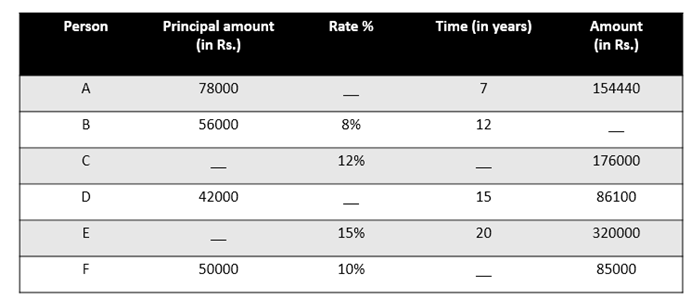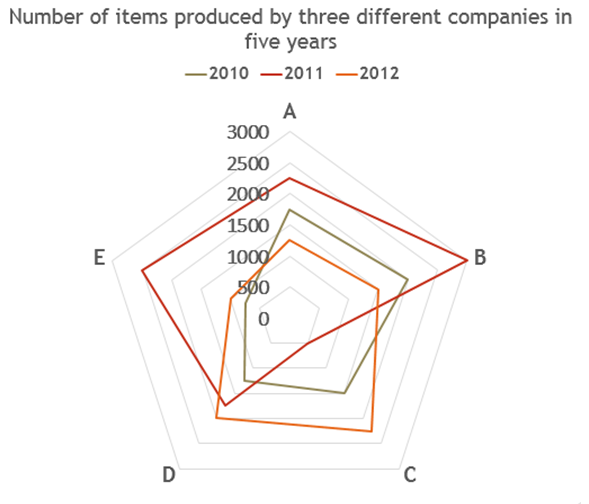# Quantitative Aptitude Questions (Data Interpretation) for SBI Clerk / IDBI Executive 2018 Day-44

Dear Readers, SBI is conducting Online preliminary Examination for the recruitment of Clerical Cadre. preliminary Examination of SBI Clerk was scheduled from June/July 2018. To enrich your preparation here we have providing new series of Data Interpretation – Quantitative Aptitude Questions. Candidates those who are appearing in SBI Clerk Prelims and IDBI Executive Exams can practice these Quantitative Aptitude average questions daily and make your preparation effective.

Click “Start Quiz” to attend these Questions and view Solutions

Directions (Q. 1 – 5): Study the following information carefully and answer the questions given below:Note: Using Simple Interest method.

1. Find the difference between rate percent of A and that of D.

a) 10.5%

b) 7%

c) 14%

d) 21%

e) None of these

1. If the amount invested by C is Rs. 110000 and the time period for which C invested is what percent of time period for which F invested.

a) 71.42%

b) 41.72%

c) 72.14%

d) 42.71%

e) None of these

3. Find the sum of the total amounts of all the persons.

a) Rs.931030

b) Rs.913300

c) Rs.931300

d) Rs.921300

e) None of these

4. Amount invested by E is what percent more than the amount invested by F?

a) 45%

b) 50%

c) 55%

d) 60%

e) None of these

1. If C invested for 10 years, find the respective ratio of amount invested by C ad that of A.

a) 49:50

b) 39:40

c) 50:49

d) 40:39

e) None of these

Directions (Q. 6 – 10): Study the following information carefully and answer the questions given below:1. Number of items produced by company A in 2010 is what percent of the number of items produced by company C in 2011?

a) 170%

b) 350%

c) 320%

d) 230%

e) None of these

1. Find the sum of total number of items produced by companies D and E in all the years together.

a) 9250

b) 9025

c) 5290

d) 5029

e) None of these

1. Number of items sold by all the companies in 2010 is what percent more than the number of items sold by company C in all the years together?

a) 57.8%

b) 75.8%

c) 70.58%

d) 50.78%

e) None of these

9. Find the difference between total number of items sold by all the companies in 2011 and 2012.

a) 2000

b) 5000

c) 3000

d) 4000

e) None of these

1. Find the respective ratio between total number of items sold by companies B, E in 2010 and total number of items sold by A and D in 2012.

a) 12:19

b) 13:11

c) 19:12

d) 11:13

e) None of these

Directions (Q. 1 – 5):

Let rate percent of A = r1%

And rate percent of D = r2%

Interest of A = 154440 – 78000 = Rs.76440

(78000 x r1 x 7)/100 = 76440

=>r1 = 7644000/(78000 x 7)

=> r1 = 14%

And

Interest of D = 86100 – 42000 = 44100

(42000 x r2 x 15)/100 = 44100

=> r2 = 4410000/(42000 x 15)

=> r2 = 7%

Required difference = 14% – 7% = 7%

Let the time for which C invested = t1 years

And the time period for which F invested = t2 years

Interest of C = 176000 – 110000 = Rs.66000

Interest of F = 85000 – 50000 = Rs.35000

(110000 x 12 x t1)/100 = 66000

=> t1 = 6600000/(110000 x 12)

=> t1 = 5 years

And

(50000 x 10 x t2)/100 = 35000

=> t2 = 3500000(50000 x 10)

=> t2 = 7 years

Required percentage = 5/7 x 100 = 71.42%

Amount of B = (56000 x 8 x 12)/100 + 56000

= 53760 + 56000

= Rs.109760

Required sum = 154440 + 109760 + 176000 + 86100 + 320000 + 85000 = Rs.931300

Let the amount invested by E = Rs.y

(y x 15 x 20)/100 + y = 320000

=> 300y + 100y = 32000000

=> 400 y = 32000000

=> y = 80000

Required percentage = (80000 – 50000)/50000 x 100 = 60%

Let the amount invested by C = Rs.y

(y  x 12 x 10)/100 + y = 176000

=> 120y + 100y = 17600000

=> 220 y = 17600000

=> y = 17600000/220

=> y = Rs.80000

Required ratio = 80000:78000 = 40:39

Directions (Q. 6 – 10):

Required percentage = (1750/500) x 100 = 350%

Required sum = 1250 + 1750 + 2000 + 750 + 2500 + 1000 = 9250

Explanation:

Number of items sold by all the companies in 2010 = 1750 + 2000 + 1500 + 1250 + 750 = 7250

Number of items sold by company C in all the years together = 1500 + 500 + 2250 = 4250

Required percentage = (7250 – 4250)/4250 x 100 = 70.58%

Total number of items sold by all the companies in 2011 = 2250 + 3000 + 500 + 1750 + 2500 = 10000

Total number of items sold by all the companies in 2012 = 1250 + 1500 + 2250 + 2000 + 1000 = 8000

Required difference = 10000 – 8000 = 2000

Total number of items sold by companies B, E in 2010 = 2000 + 750 = 2750

Total number of items sold by companies A and D in 2012 = 1250 + 2000 = 3250

Required ratio = 2750 : 3250 = 11:13

Daily Practice Test Schedule | Good Luck

 Topic Daily Publishing Time Daily News Papers & Editorials 8.00 AM Current Affairs Quiz 9.00 AM Logical Reasoning 10.00 AM Quantitative Aptitude “20-20” 11.00 AM Vocabulary (Based on The Hindu) 12.00 PM Static GK Quiz 1.00 PM English Language “20-20” 2.00 PM Banking Awareness Quiz 3.00 PM Reasoning Puzzles & Seating 4.00 PM Daily Current Affairs Updates 5.00 PM Data Interpretation / Application Sums (Topic Wise) 6.00 PM Reasoning Ability “20-20” 7.00 PM English Language (New Pattern Questions) 8.00 PM General / Financial Awareness Quiz 9.00 PM